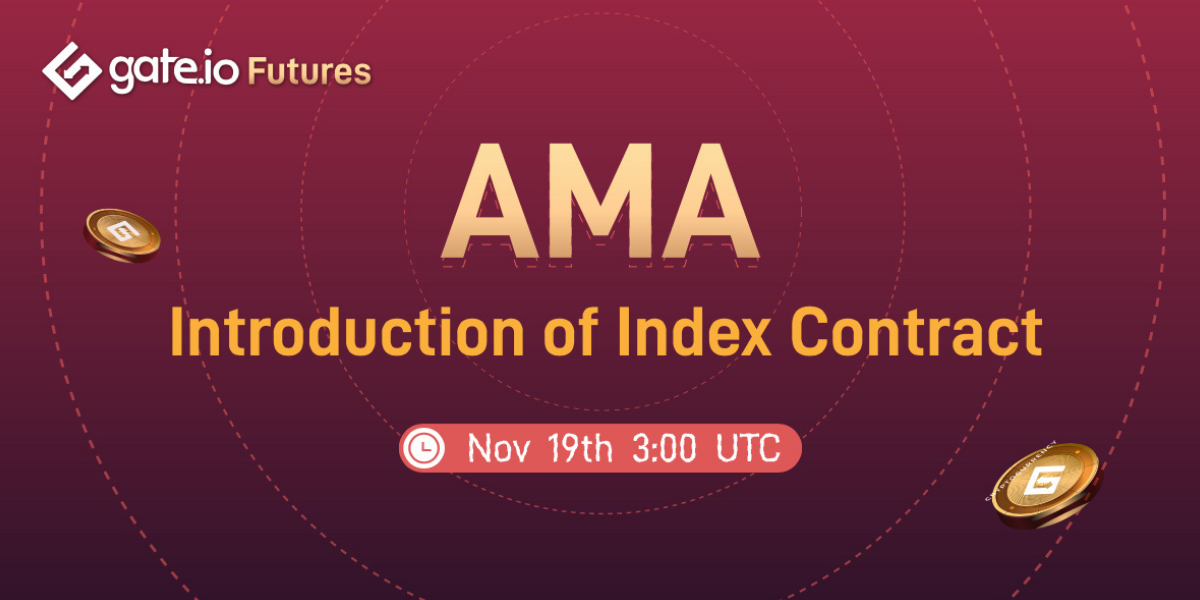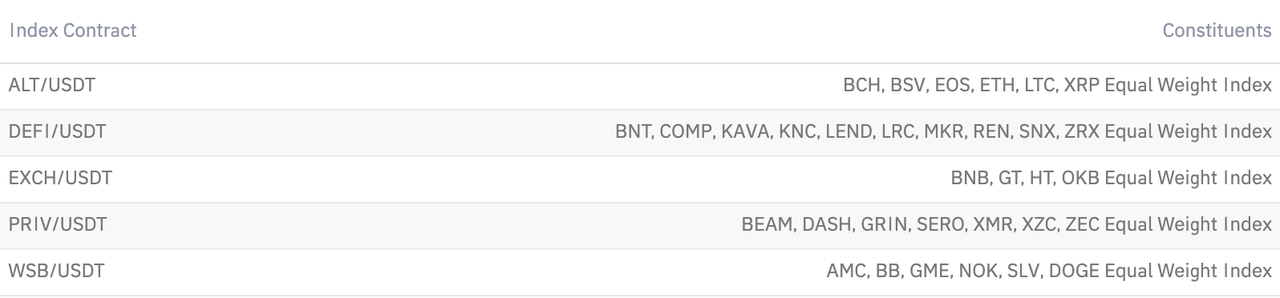• Preference Settings
From- To time of the change range
Theme
Bright
Classic black
Pitch black
• Exchange Rate Switch
Language
• 简体中文
• English
• Tiếng Việt
• 繁体中文
• Español
• Русский язык
• Français
• Deutsch
• Italiano
• Português
• ภาษาไทย
• Indonesia
• Türkçe
• 日本語
Exchange Rate Switch
• CNY
• USD
• KRW
• VND
• EUR
• GBP
• HKD
• JPY
• RUB
• TRY
• INR
Gate.io Blog

• Gate Blog
• Options
• Guide
• Dialogues
AMA 101

# Introduction of Index Contract

23 November 18:33A : An index contract is a contract based on the equal weight index.

The equal-weight index gives each component token the exact same weight and ensures it through regular adjustment.

For example:

An equal-weight index is divided into A, B, C, and D 4 types of tokens with the price of 1, 2, 5, 10USDT,

Buy 2,000USDT index, then the price of the purchase of every token is 500USDT, the amount of every token is 500, 250, 100, 50.

If token A rises by 10%, token B falls by 5%, token C falls by 2%, token D rises by 3%, the increase of the index is:(10%-5%-2%+3%)/4=1.5%.

The rise and fall of each token have the same impact on the index so that it's called an Equal Weight Index.

In the beginning, every component token has the same weight, but the weight changes with the rise and fall of each component token and will no longer be the same. That the equal-weight index will be force balanced each quarter.

Q : Any Advantages of Index Contract ?

A : Compared with the single currency index contract, the index contract has the following advantages:

1)It's easier to make choices, only need to select a track.

Take the popular DEFI as an example, which includes dozens of tokens of which most people do not have time to do any research. In this case, it's difficult to select a token with investment value.

An investment index contract makes all these much easier. Users can simply buy the DEFI Index Contract if they think it will develop well in the future.

2)Diversify investment risks

Compared with the rise and fall of a single token, the rise and fall of the index will be smaller, which will smooth the boom and bust of some tokens and provide enough time to stop loss.

Q : Can you tell me about each index contract ?

1).ALT_USDT

Consist of the token with great market value and liquidity, which generally reflect the trend of the crypto market.

2).EXCH_USDT

Consist of the tokens of mainstream exchanges, which reflect the development of the exchanges.

3).DEFI_USDT

Consist of the DEFI tokens with great liquidity and potential, which reflect the development of the DEFI industry.

4)PRIV_USDT

Consist of common anonymous tokens, which reflect the development of the anonymous token market.

5)WSB_USDT

Consist of tokenized stock like AMC Entertainment Holdings (AMC), BlackBerry (BB), GameStop (GME), Nokia (NOK), iShares Silver Trust (SLV) andDOGE tokens, which reflect the trend of the hotspot of Wall Street.

Components of each Equal-weight Index：Q : How about Index Rebalance ?

A : Rebalancing refers to the mechanism by which the token distribution will need to be adjusted to equal weight after a market fluctuation.

In the process of adjustment, the equal weight will be reduced if the proportion of previous high-increase component tokens is higher than the average proportion; and vise versa.

Example:

A : An equal-weight index includes A, B, C, D 4 types of tokens with the price of 1, 2, 5, 10USDT and an initial index at 1000. The share of each index is an average of 25%, 250 shares. The corresponding equal weight is 250, 125.5, 50, 25.

After a period of time, the price of the 4 tokens is 1.2, 3.2, 5.2, 8 with an index of 1,191.6 and the share of each token is 25.18%, 33.70%, 23.24%, 16.78%.That indicates token B with a higher rise which exceeds much than 25% and token D with a higher fall which exceeds much than 25%.

Under this circumstance, the rebalancing will be triggered and adjust the proportions of all 4 types of tokens to 25% with an average share of 297.9 and corresponding weight at 248.25, 93.09, 51.36, 37.24.

Compared with the previous weight, it's easy to tell the weight of a token with a higher rise will reduce, such like token A and B; and the weight of a token with a lower rise or fall will increase, such as token C and D.

Note: The index value refers to each component token price multiplied with its weight and the get the sum of all.

Like the example mentioned above, the index value is:

1191.6 = 1.2 * 250 + 3.2 * 125.5 + 5.8 * 50 + 8 * 25

In the process of rebalancing, the adjusted weight of the token equals its share divided by the price.

Like the example mentioned above, the rebalanced weight of token A is:

248.25 = 297.9 / 1.2

In the actual process of rebalancing, in order to smooth the transition, the weight is not adjusted in place at once but gradually adjusted over time.

During this period of time, the weight can be adjusted by a certain range and at the same amount.

The calculation of each taken weight :

Smooth Weight = ( Target Weight - Original Weight ) / Duration Time of Adjustment * Accumulated Adjustment Time + Original Weight

Instructions of the formula:

*Smooth Weight: The transition weight during the rebalancing process is used for the smooth index, which equals the target weight after the rebalancing.

*Target Weight: The weight calculated during rebalancing. Like the example mentioned above, the target weight of token A is 248.25, and the target weight of token B is 93.09.

*Original Weight: The weight before rebalancing. Like the example mentioned above, the original weight of token A is 250, and the original weight for token B is 125.5.

*Duration Time of Adjustment: the length of adjustment time, like '1 hour'.

*Accumulated Adjustment Time: The smooth weight is calculated every interval of time, like every 10 seconds. The time value will increase with every calculation. Assuming that the duration of adjustment time is 1 hour, which is 3,600 seconds. While the smooth weight is calculated every 10 seconds, the accumulated adjustment time will gradually increase from 0, 10, 20 to 3,600.

Rebalancing Time of Index：

A : The contract index is rebalanced quarterly. The specific date and time are the 28th of every March, June, September, and December at 0:00 (TGE+8).

The adjustment will last for one hour and the smooth weight will be calculated every 10 seconds.

A : That's all for today. Let's move to the question sessions.

Share to：
Trending
09 November 15:35
02 November 17:24
12 November 16:58
15 November 18:40